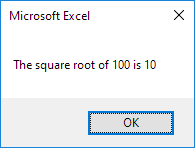# Type Mismatch

The type mismatch error in Excel VBA occurs when you try to assign a value to a variable that isn't of the correct type.

Place a command button on your worksheet and add the following code lines:

Dim number As Integer
number = "bike"

Result when you click the command button on the sheet: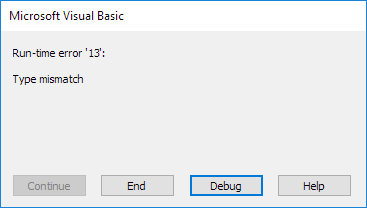Explanation: you cannot assign a string value (bike) to a variable of type Integer.

The type mismatch error (run-time error 13) often occurs when using the InputBox function in Excel VBA.

1. Place a command button on your worksheet and add the following code lines:

Dim number As Integer
number = InputBox("Enter a number", "Square Root")

MsgBox "The square root of " & number & " is " & Sqr(number)

2. Click the command button on the sheet, enter the string value hundred and click OK.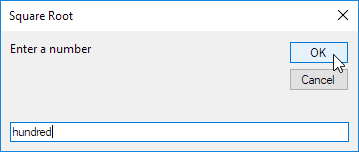Result:3. Clik End.

4. To display a friendly message instead of the type mismatch error, update the code as follows.

Dim number As Variant
number = InputBox("Enter a number", "Square Root")

If IsNumeric(number) Then
MsgBox "The square root of " & number & " is " & Sqr(number)
Else
End If

Explanation: a variable of type Variant can hold any type of value (see first line). The IsNumeric function in Excel VBA checks if a value is a number.

5. Repeat step 2.

Result: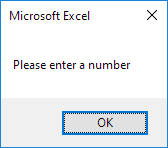6. Finally, check if this simple square root calculator works.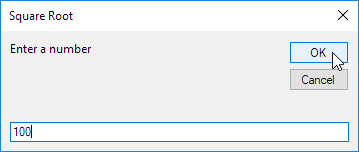Result: